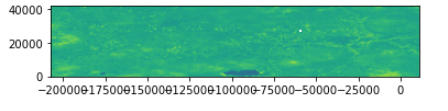# Save original projection of image

Hello,

I’m trying to understand better how the projection works with the images I download.
When I use rasterio image it keeps the coordinates system data per pixel so I can plot my shapefile on top of it.
When I use the plot_image function I don’t see the coordinates, and any activity I do later with the image is as numpy and then I lose to CRS.
I s there any way to save the CRS data for the image so I can reprojec tthe image after working on it as numpy array?

Best

Reut

1 Like

Hi @reutkeller,

Here are a couple of suggestions:

• If you download an image from Sentinel Hub service in tiff format the image will be georeferenced. If you download using `sentinelhub-py` you have an option to save data as shown in this example. This will save the original georeferenced tiff image.

• In memory, `sentinelhub-py` decodes an image into a normal `numpy` array without geoinformation. But you also have an instance of `sentinelhub.BBox` object which was used to request an image from Sentinel Hub service and it defines coordinates and CRS of a downloaded image. Therefore you can collect geo information from it and pass it to `rasterio` functions. For that the following methods should be useful:

I hope this helps.

1 Like

so now , i’m trying the BBox.crs.pyproj_crs but not sure how to use it as when I run it I get:

``````bbox.crs.pyproj_crs

>>>
<bound method CRS.pyproj_crs of CRS('4326')>
``````

and ofcurse when I try to save it as raster with rasterio it doesn’t understand it:

``````import rasterio
from rasterio.transform import from_origin
transform=from_bounds(minx,maxy,pixel_size,pixel_size,ndvi.shape,ndvi.shape)
#crs_img='EPSG:4326'

with rasterio.open('test1.tif',
'w',
driver='GTiff',
height=ndvi.shape,
width=ndvi.shape,
count=1,
dtype=ndvi.dtype,
nodata=None, # change if data has nodata value
transform=transform) as dst:
dst.write(ndvi, 1)

>>>

CRSError: CRS is invalid: <bound method CRS.pyproj_crs of CRS('4326')>
``````

The `pyproj_crs` is a method, so you should use `pyproj_crs()`.

Cheers,
Matej

1 Like

Thank you.
and just to make sure I understood correct,

in bbox.get_transform_vector() , How do I know what is the resx and resy? is it based on the image resolution? e.g if I use sentinel2 it will be 10,10 , or landsat will be 30,30…

I’m asking because when I do it as you mentioned I get weird image:
original image:

``````img_crs=bbox.crs.pyproj_crs()

transform=bbox.get_transform_vector(10,10)

transform=rasterio.Affine(-101.7359960059834, 10.0, 0, 20.8312118894487, 0, -10.0) #this equal to the tuple from get_transform_vector

with rasterio.open('test1.tif',
'w',
driver='GTiff',
height=ndvi.shape,
width=ndvi.shape,
count=1,
dtype=ndvi.dtype,
crs=img_crs,
nodata=None, # change if data has nodata value
transform=transform) as dst:
dst.write(ndvi, 1)

``````

when I open this image I get this:so i’m not sure if the problem is resx resy or osmething else.

Thank you for the help

Reut

Be careful of the units. You data is in WGS84 (units are degrees), while you trying to use the resolution in metres.

I have tried to insert the degrees, as I understand, if I use sentinel1image now with 10 meters resolution it should look like this:

``````trans=bbox.get_transform_vector(0.00009009009009,0.00009009009009)
``````

but still I get it wrong . I believe I miss understood you regard the meters and degrees?

To be honest, I’d be using eo-learn, where ExportToTiff functionality takes care of all these issues.

But if you have a quick look at that code, you will see that it uses width and height (as opposed to resx/resy):

``````crs = bbox.crs.pyproj_crs()
transform = rasterio.transform.from_bounds(*bbox, width=width, height=height)
``````

Perhaps you could try that?

Hi,
I am not trying to push my own writing, but perhaps this blog helps you:
https://towardsdatascience.com/geo-referencing-a-figure-as-an-image-fc5e77caa00b

In the post, I try to explain how to handle different coordinate systems, and what the effect of the transformation is.

Kind regards,
Gijs

1 Like